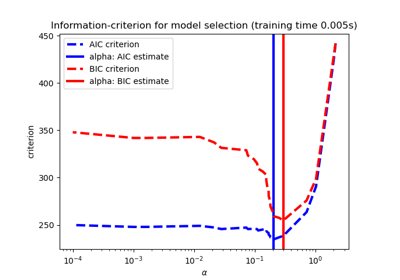# 3.2.4.2.1. sklearn.linear_model.LassoLarsIC¶

class sklearn.linear_model.LassoLarsIC(criterion='aic', *, fit_intercept=True, verbose=False, normalize=True, precompute='auto', max_iter=500, eps=2.220446049250313e-16, copy_X=True, positive=False)[source]

Lasso model fit with Lars using BIC or AIC for model selection

The optimization objective for Lasso is:

(1 / (2 * n_samples)) * ||y - Xw||^2_2 + alpha * ||w||_1


AIC is the Akaike information criterion and BIC is the Bayes Information criterion. Such criteria are useful to select the value of the regularization parameter by making a trade-off between the goodness of fit and the complexity of the model. A good model should explain well the data while being simple.

Read more in the User Guide.

Parameters
criterion{‘bic’ , ‘aic’}, default=’aic’

The type of criterion to use.

fit_interceptbool, default=True

whether to calculate the intercept for this model. If set to false, no intercept will be used in calculations (i.e. data is expected to be centered).

verbosebool or int, default=False

Sets the verbosity amount

normalizebool, default=True

This parameter is ignored when fit_intercept is set to False. If True, the regressors X will be normalized before regression by subtracting the mean and dividing by the l2-norm. If you wish to standardize, please use sklearn.preprocessing.StandardScaler before calling fit on an estimator with normalize=False.

precomputebool, ‘auto’ or array-like, default=’auto’

Whether to use a precomputed Gram matrix to speed up calculations. If set to 'auto' let us decide. The Gram matrix can also be passed as argument.

max_iterint, default=500

Maximum number of iterations to perform. Can be used for early stopping.

epsfloat, optional

The machine-precision regularization in the computation of the Cholesky diagonal factors. Increase this for very ill-conditioned systems. Unlike the tol parameter in some iterative optimization-based algorithms, this parameter does not control the tolerance of the optimization. By default, np.finfo(np.float).eps is used

copy_Xbool, default=True

If True, X will be copied; else, it may be overwritten.

positivebool, default=False

Restrict coefficients to be >= 0. Be aware that you might want to remove fit_intercept which is set True by default. Under the positive restriction the model coefficients do not converge to the ordinary-least-squares solution for small values of alpha. Only coefficients up to the smallest alpha value (alphas_[alphas_ > 0.].min() when fit_path=True) reached by the stepwise Lars-Lasso algorithm are typically in congruence with the solution of the coordinate descent Lasso estimator. As a consequence using LassoLarsIC only makes sense for problems where a sparse solution is expected and/or reached.

Attributes
coef_array-like of shape (n_features,)

parameter vector (w in the formulation formula)

intercept_float

independent term in decision function.

alpha_float

the alpha parameter chosen by the information criterion

n_iter_int

number of iterations run by lars_path to find the grid of alphas.

criterion_array-like of shape (n_alphas,)

The value of the information criteria (‘aic’, ‘bic’) across all alphas. The alpha which has the smallest information criterion is chosen. This value is larger by a factor of n_samples compared to Eqns. 2.15 and 2.16 in (Zou et al, 2007).

Notes

The estimation of the number of degrees of freedom is given by:

“On the degrees of freedom of the lasso” Hui Zou, Trevor Hastie, and Robert Tibshirani Ann. Statist. Volume 35, Number 5 (2007), 2173-2192.

Examples

>>> from sklearn import linear_model
>>> reg = linear_model.LassoLarsIC(criterion='bic')
>>> reg.fit([[-1, 1], [0, 0], [1, 1]], [-1.1111, 0, -1.1111])
LassoLarsIC(criterion='bic')
>>> print(reg.coef_)
[ 0.  -1.11...]


Methods

 fit(X, y[, copy_X]) Fit the model using X, y as training data. get_params([deep]) Get parameters for this estimator. Predict using the linear model. score(X, y[, sample_weight]) Return the coefficient of determination R^2 of the prediction. set_params(**params) Set the parameters of this estimator.
__init__(criterion='aic', *, fit_intercept=True, verbose=False, normalize=True, precompute='auto', max_iter=500, eps=2.220446049250313e-16, copy_X=True, positive=False)[source]

Initialize self. See help(type(self)) for accurate signature.

fit(X, y, copy_X=None)[source]

Fit the model using X, y as training data.

Parameters
Xarray-like of shape (n_samples, n_features)

training data.

yarray-like of shape (n_samples,)

target values. Will be cast to X’s dtype if necessary

copy_Xbool, default=None

If provided, this parameter will override the choice of copy_X made at instance creation. If True, X will be copied; else, it may be overwritten.

Returns
selfobject

returns an instance of self.

get_params(deep=True)[source]

Get parameters for this estimator.

Parameters
deepbool, default=True

If True, will return the parameters for this estimator and contained subobjects that are estimators.

Returns
paramsmapping of string to any

Parameter names mapped to their values.

predict(X)[source]

Predict using the linear model.

Parameters
Xarray_like or sparse matrix, shape (n_samples, n_features)

Samples.

Returns
Carray, shape (n_samples,)

Returns predicted values.

score(X, y, sample_weight=None)[source]

Return the coefficient of determination R^2 of the prediction.

The coefficient R^2 is defined as (1 - u/v), where u is the residual sum of squares ((y_true - y_pred) ** 2).sum() and v is the total sum of squares ((y_true - y_true.mean()) ** 2).sum(). The best possible score is 1.0 and it can be negative (because the model can be arbitrarily worse). A constant model that always predicts the expected value of y, disregarding the input features, would get a R^2 score of 0.0.

Parameters
Xarray-like of shape (n_samples, n_features)

Test samples. For some estimators this may be a precomputed kernel matrix or a list of generic objects instead, shape = (n_samples, n_samples_fitted), where n_samples_fitted is the number of samples used in the fitting for the estimator.

yarray-like of shape (n_samples,) or (n_samples, n_outputs)

True values for X.

sample_weightarray-like of shape (n_samples,), default=None

Sample weights.

Returns
scorefloat

R^2 of self.predict(X) wrt. y.

Notes

The R2 score used when calling score on a regressor uses multioutput='uniform_average' from version 0.23 to keep consistent with default value of r2_score. This influences the score method of all the multioutput regressors (except for MultiOutputRegressor).

set_params(**params)[source]

Set the parameters of this estimator.

The method works on simple estimators as well as on nested objects (such as pipelines). The latter have parameters of the form <component>__<parameter> so that it’s possible to update each component of a nested object.

Parameters
**paramsdict

Estimator parameters.

Returns
selfobject

Estimator instance.

## 3.2.4.2.1.1. Examples using sklearn.linear_model.LassoLarsIC¶